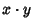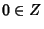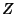## Tame Algebra

Letdenote an-algebra, so thatis a Vector Space overandwhereis vector multiplication which is assumed to be Bilinear. Now definewhere.is said to be tame ifis a finite union of Subspaces of. A 2-D 0-Associative algebra is tame, but a 4-D 4-Associative algebra and a 3-D 1-Associative algebra need not be tame. It is conjectured that a 3-D 2-Associative algebra is tame, and proven that a 3-D 3-Associative algebra is tame if it possesses a multiplicative Identity Element.

References

Finch, S. Zero Structures in Real Algebras.'' http://www.mathsoft.com/asolve/zerodiv/zerodiv.html.